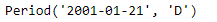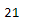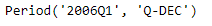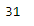Python | Pandas Period.day

• Last Updated : 06 Jan, 2019

Python is a great language for doing data analysis, primarily because of the fantastic ecosystem of data-centric python packages. Pandas is one of those packages and makes importing and analyzing data much easier.

Pandas Period.day attribute returns the day of the month for the given Period object. It returns an integer value.

Syntax : Period.day

Parameters : None

Return : day

Example #1: Use Period.day attribute to find the day in the given Period object.

 # importing pandas as pdimport pandas as pd  # Create the Period objectprd = pd.Period(freq ='D', year = 2001, month = 1, day = 21)  # Print the Period objectprint(prd)

Output :Now we will use the Period.day attribute to find the day of the month in the given object.

 # return the day value prd.day

Output :As we can see in the output, the Period.day attribute has returned 21 which is the value of day in the given period object.

Example #2: Use Period.day attribute to find the day in the given Period object.

 # importing pandas as pdimport pandas as pd  # Create the Period objectprd = pd.Period(freq ='Q', year = 2006, quarter = 1)  # Print the objectprint(prd)

Output :Now we will use the Period.day attribute to find the day of the month in the given object.

 # return the day value prd.day

Output :As we can see in the output, the Period.day attribute has returned 31 which is the value of day in the given period object.

My Personal Notes arrow_drop_up